#Picard Iterative ProcessIndeed, often it is very hard to solve differential equations, but we do have a numerical process that can approximate the solution. This process is known as the Picard iterative process.
First, consider the IVP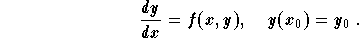It is not hard to see that the solution to this problem is also given as a solution to (called the integral associated equation)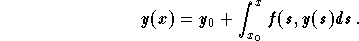The Picard iterative process consists of constructing a sequence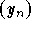of functions which will get closer and closer to the desired solution. This is how the process works:

(1)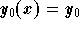for every x;
(2)
then the recurrent formula holds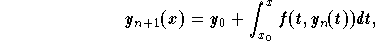for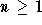.

Example: Find the approximated sequence, for the IVP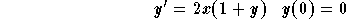.

Solution: First let us write the associated integral equation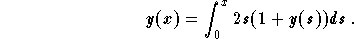Set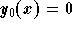. Then for any, we have the recurrent formula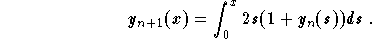We have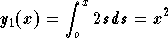, and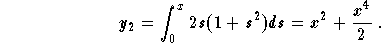We leave it to the reader to show that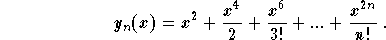We recognize the Taylor polynomials of (which also get closer and closer to) the function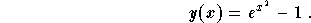[Differential Equations] [First Order D.E.]
[Geometry] [Algebra] [Trigonometry ]
[Calculus] [Complex Variables] [Matrix Algebra]S.O.S MATHematics home page

Do you need more help? Please post your question on our S.O.S. Mathematics CyberBoard.Author: Mohamed Amine Khamsi